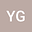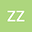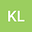Asymptotic estimations of eigenvalues and eigenfunctions for Nonlocal Boundary Value Problems with eigenparameter-dependent boundary conditions
•••• Yu Gan,
• Zhaowen Zheng,
• Kun Li
Yu Gan
Qufu Normal University School of Mathematical Sciences

Corresponding Author:1774320591@qq.om

Author ProfileZhaowen Zheng
Qufu Normal University School of Mathematical Sciences
Author ProfileKun Li
Qufu Normal University
Author Profile## Abstract

The nonlocal boundary value problem with eigenparameter dependent boundary conditions is studied in this paper. Firstly, we give the asymptotic expressions of the general solution for the equation corresponding to the initial conditions with eigenparameters, then we prove the multiplicity of eigenvalues some properties of the eigenvalues and eigenfunctions. Finally, the asymptotic formulas of eigenvalues and eigenfunctions are obtained under certain mild conditions. Our method is to incorporate the perturbation theory and asymptotic analysis in the framework of classical Sturm-Liouville problems, which provides a new sight for the investigating of the Sturm-Liouville problems with eigenparameter in boundary conditions.